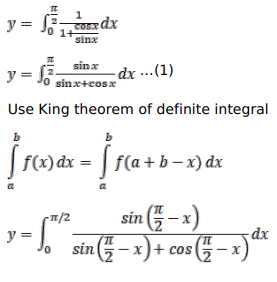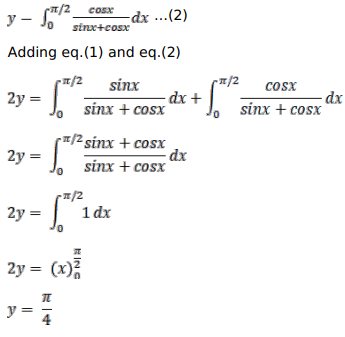# Solve this followingQuestion:

Prove that

$\int_{0}^{\pi / 2} \frac{\mathrm{dx}}{(1+\cot \mathrm{x})}=\frac{\pi}{4}$

Solution: